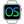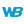HelpWLanguageWLanguage syntaxType of dataAdvanced typesThe different types of integersTypes of integersNotesSee alsoAlias typeBufferCharacter stringsConverting C typesCurrenciesDateDateTimeDifferent types of dataDurationJSONNumericOperations available for the dates, times and durations, ...RealsRectangleThe different types of integersTimeUUIDVariantYAML
• Types of integers
• Different types of integers are available in WLanguage
• Notes
• Default value
• Thousand separator
• Specific features of PHP
• Differences between WINDEV 5.5 and WINDEV 27
WINDEVWEBDEVWINDEV MobileOthersThe different types of integers
Types of integers

Different types of integers are available in WLanguage

 Value included between Integer -2 x 109 and 2 x 109 (roughly)Example: i is inti = 5 1-byte integer -128 and 127 (inclusive)Example: i is 1-byte int 2-byte integer -32.768 and 32.767 (inclusive)Example: I is 2-byte int 4-byte integer -2 x 109 and 2 x 109 (roughly)Example: i is 4-byte int 8-byte integer -9 x 1018 and 9 x 1018 (roughly)Example: i is 8-byte int Unsigned integer 0 and 4 x 109Example: i is unsigned int Byte 0 and 255 (inclusive)This type is equivalent to the unsigned 1-byte integer.Example: i is byte Unsigned 1-byte integer 0 and 255 (inclusive)Example: i is unsigned 1-byte int Unsigned 2-byte integer 0 and 65.535 (inclusive)Example: i is unsigned 2-byte int Unsigned 4-byte integer 0 and 4 x 109 (roughly)Example: i is unsigned 4-byte int Unsigned 8-byte integer 0 and 18 x 1018Example: i is unsigned 8-byte int System integer automatically adapts to the size supported by the compilation mode (4 bytes for a program compiled in 32 bits, 8 bytes for a program compiled in 64 bits). Example: i is system intRemark: SystemIntegerToInteger is used to convert a system integer into a 4-byte integer.
The exact values for these different types of integers can be found in the Limits.wl file found in the Personal\External subdirectory of the setup directory of WINDEV, WEBDEV and WINDEV Mobile.
Remark: All the advanced types (different from the "Integer" type) are recommended when using the Windows APIs.
Notes

Default value

An "Integer" variable that is declared but not initialized is equal to 0.

To enter an hexadecimal value in the code, the hexadecimal value must be prefixed by "0x".
MyVariable is int
MyVariable = 0x4D5
Remark: The '_' character can be used as block separator in the hexadecimal numbers. The blocks can have a size equal to 2, 4 or 8 characters long.
Example:
Nb1 is int = 0x_12_15_A4_BC
Nb2 is int = 0x_CD4A_DE58

Thousand separator

You can use spaces and underscore characters to separate thousands in numbers. For example:
num1 is int
num1 = 123 456
let num2 = 246_789Specific features of PHP

• Numbers (real, integer, currency, numeric) cannot exceed 14 significant digits in PHP. If they exceed this limit, they are rounded to 14 digits.
• The integers in 32 bits have for maximum value:
2147483647 for 32 bits <=> 0x7FFFFFFF
• The integers in 64 bits have for maximum value:
9223372036854775807<=>0x7FFFFFFFFFFFFFFFDifferences between WINDEV 5.5 and WINDEV 27

The types of integers have been modified between WINDEV 5.5 and WINDEV 27:
Type of integers in WINDEV 5.5 Type of integers in WINDEV 27 Integer 2-byte integer Unsigned integer Unsigned 2-byte integer Long integer 4-byte integer Short integer Unsigned 1-byte integer
In WINDEV 5.5, integers were 2-byte integers by default. Since the versions 7.x, integers are 4-byte integers by default.
Minimum version required
• Version 9